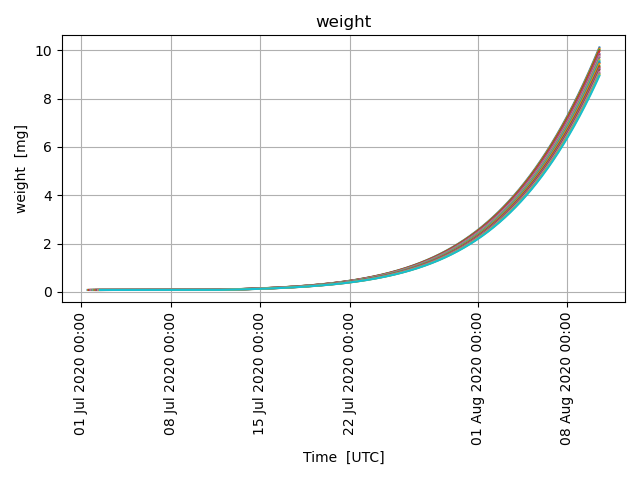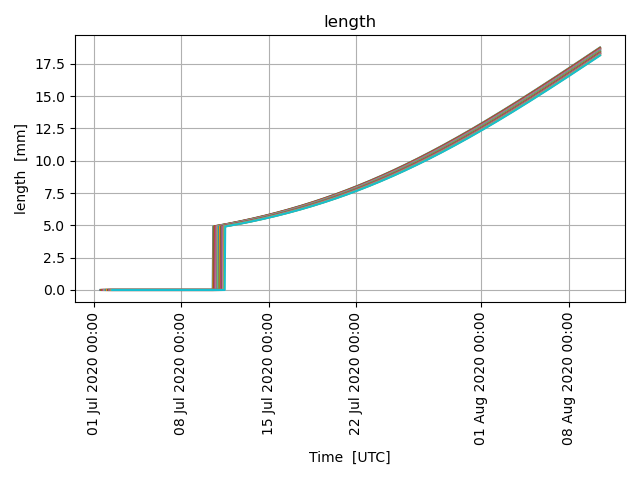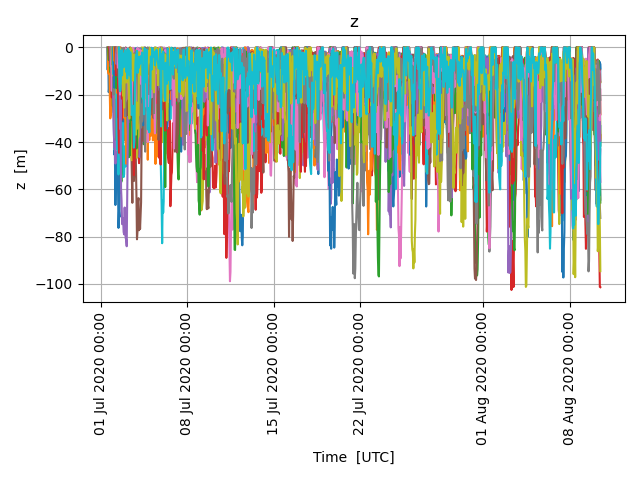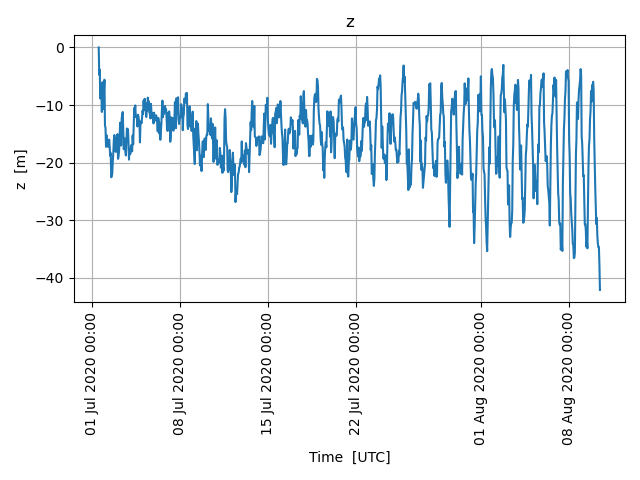# Fish Eggs and Larvae

```from datetime import datetime, timedelta
from opendrift.models.larvalfish import LarvalFish

o = LarvalFish(loglevel=50)
```

Seeding 20 fish eggs, which will hatch as larvae after some time, depending om temperature

```time = datetime(2020, 7, 1, 12)
o.seed_elements(lon=4, lat=60, time=[time, time+timedelta(hours=24)], number=20)
```

No horizontal movement, here only investigating vertical mixing and swimming

```r = ConstantReader(
{'x_sea_water_velocity': 0, 'y_sea_water_velocity': 0, 'x_wind': 0, 'y_wind': 0,
'sea_water_temperature': 10,

o.run(duration=timedelta(days=40))
```

After 20 days eggs are hatched as Larvae, and starting to grow

```o.plot_property('weight')
o.plot_property('length')
```
••We see that larvae (after hatching) avoid the upper meters at daytime, to avoid predators. Vertical motion is a combination of vertical mixing, buoyancy and swimming.

```o.plot_property('z')
```Mean daily depth shows more clearly the diurnal migration of larvae

```o.plot_property('z', mean=True)
```Total running time of the script: (0 minutes 28.768 seconds)

Gallery generated by Sphinx-Gallery